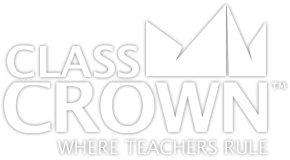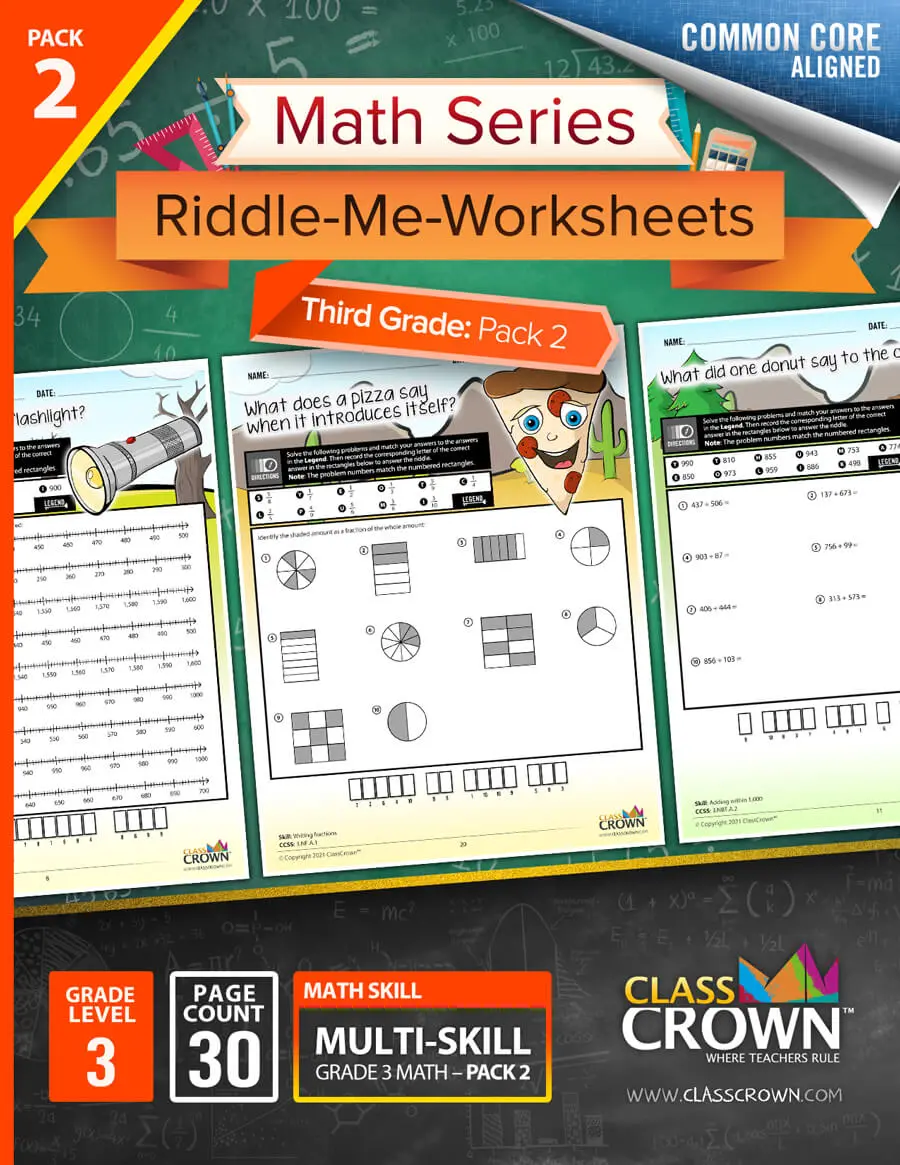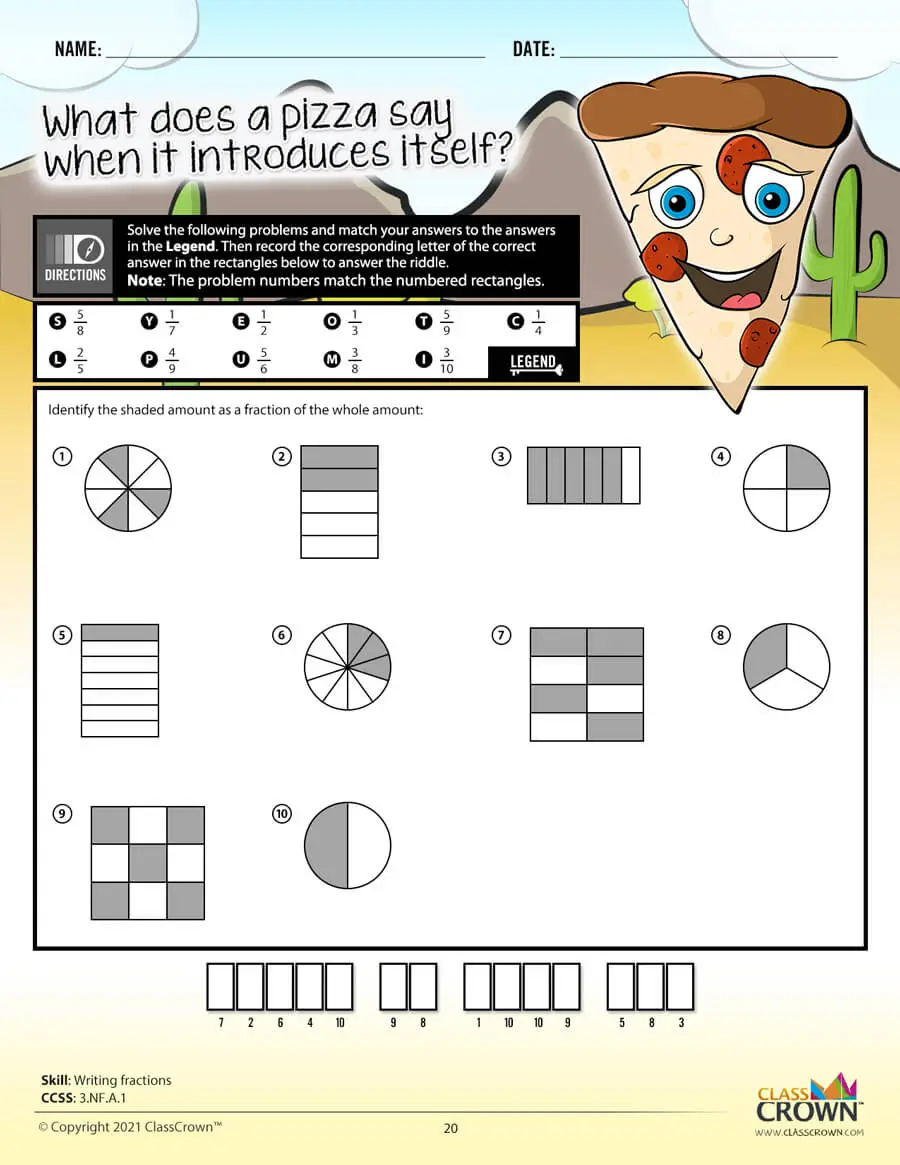Math Worksheets

# 3rd Grade Math Worksheets: Pack 2

## Self-checking math worksheets with riddles to keep kids motivated.

Rating: 0/5
(0 Reviews)
3rd
WORKSHEET COUNT
30
FORMAT(S)
PDF
Yes
\$8.99
This comprehensive bundle includes 3rd grade packs 1–3.
\$20.99
\$26.97
Save 22%
The Details
Description
• Do your students ever complete an entire math worksheet, only to find that they have done most of the problems incorrectly and clearly have a misconception?
• ClassCrown’s Riddle-Me-Worksheets help to prevent this problem by introducing a puzzle aspect to math, giving students immediate feedback as to whether or not they are solving problems correctly. If the answer to the riddle isn't correct, the student knows which problems he's made an error on.
Specifics
• Subject:  Fractions, Rounding, Number Lines +more!
• Product Count:  30
• Full Page Count:  51
• File Format(s):  PDF

What's Included?
• Estimating products
• Finding rounding amount
• Rounding to the nearest hundred with number lines
• Rounding to the nearest tens with number lines
• Rounding to tens and hundreds
• Subtracting within 1,000
• Adding and subtracting within 1,000
• Adding and subtracting within 1,000 with variables
• Subtracting across zero
• Multiplying multiples of 10
• Determining fractions (visual)
• Identifying fractions
• Writing fractions
• Subtracting fractions
• Finding fractions on a number line
• Finding equivalent fractions with number lines
• Comparing fractions
• Finding equivalent fractions
• Creating equivalent fractions with whole numbers
• Expressing whole numbers as fractions
• Expressing fractions as whole numbers
• Identify whole numbers as fractions
• Comparing fractions (same numerator and denominator)
• Comparing fractions (visual)
• Determining zero, half or whole
• Ordering fractions
• Comparing fractions
CCSS: 3.NBT.A.1, 3.NBT.A.2, 3.NBT.A.3, 3.NF.A.1, 3.NF.A.2A, 3.NF.A.3A, 3.NF.A.3B, 3.NF.A.3C, 3.NF.A.3D
Reviews
Overall Rating: 0/5
(Showing 0 of 0)
See More Reviews @ TpTSelf Checking

Our math worksheets introduce a puzzle aspect to math, giving students immediate feedback as to whether or not they are solving problems correctly. If the answer to the riddle isn't spelled correctly, the student knows which problems he's made an error on.Fun Puzzle AspectImmediate Feedback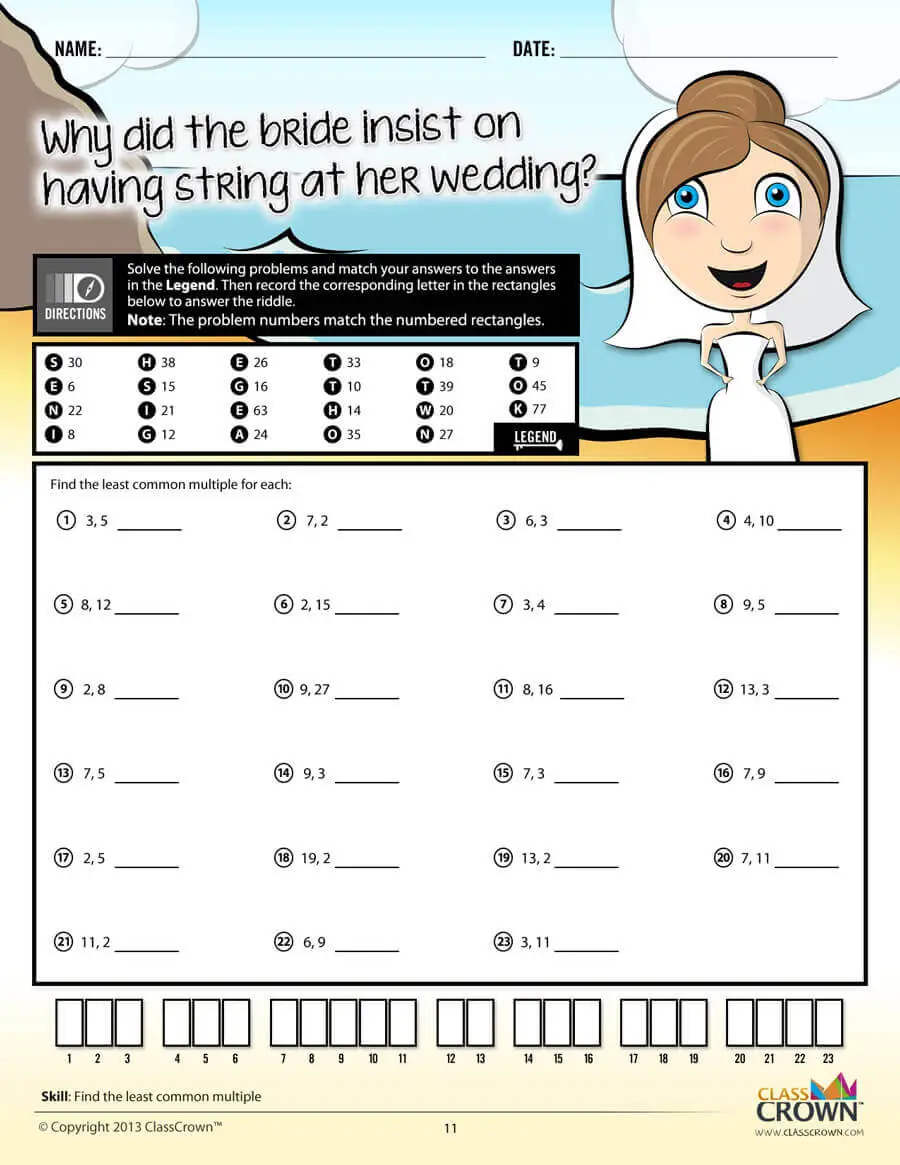Problem Solving Motivation

Each math worksheet contains a riddle that the student solves by completeing all the problems on the worksheet. This keeps kids motivated to complete each problem so that they can find the answer to the riddle.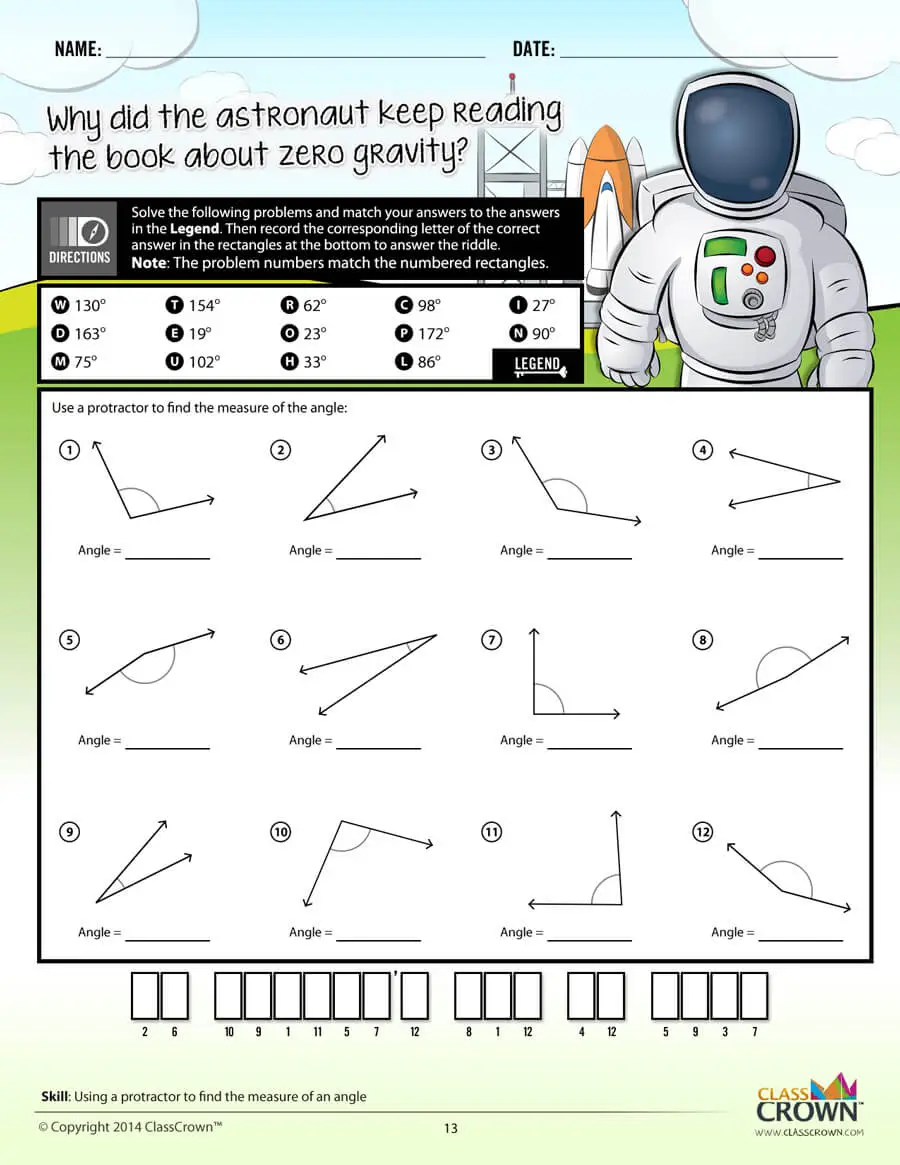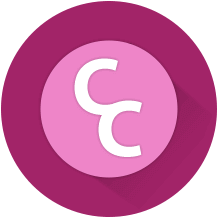Common Core Aligned

All our math worksheet packs are designed with Common Core in mind. That way you don’t have to worry about whether your math ciriculum is aligned or not when you incorpoate ClassCrown Riddle-Me-Worksheets in your lesson plans.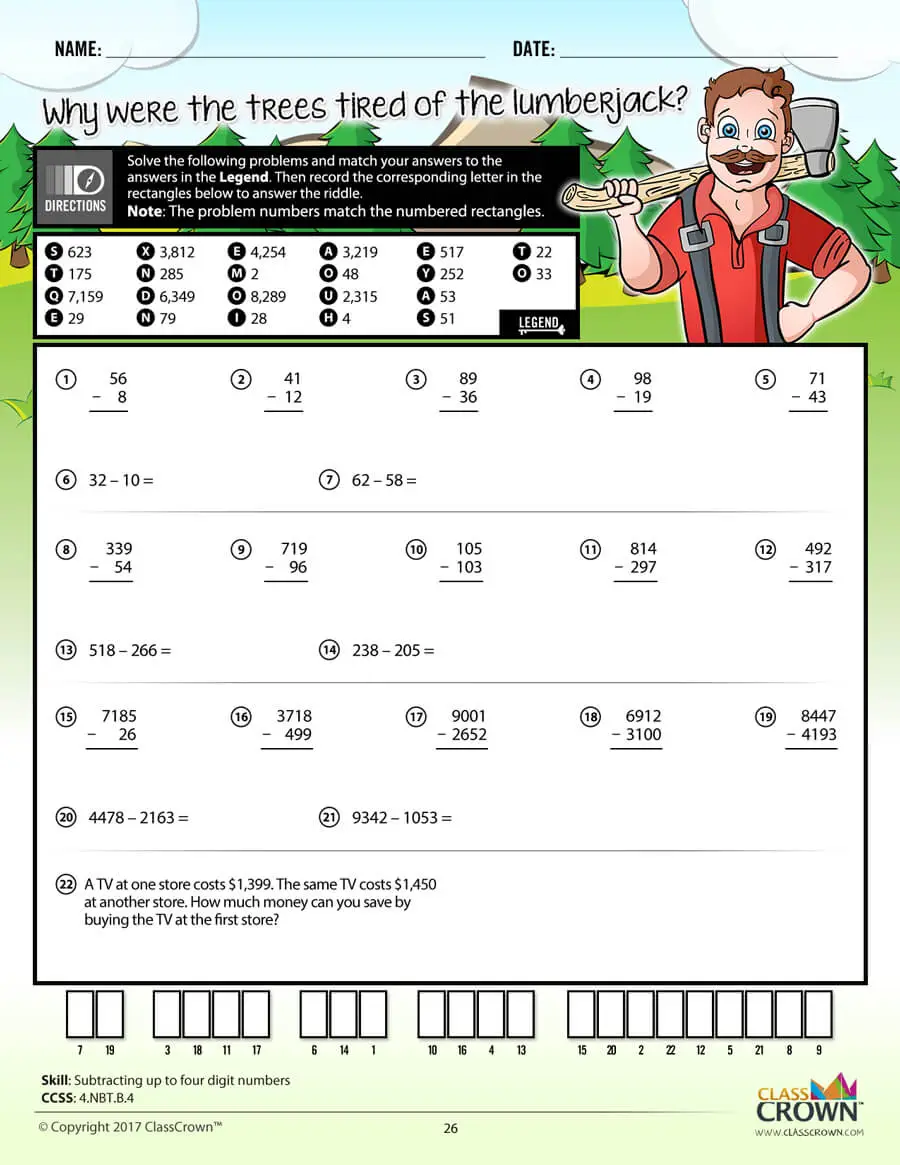High Quality Design

Each page of our math worksheets has been produced in high resolution at 144 dpi and designed in full, vibrant color for maximum quality. They look stunning whether you are printing in color or black and white.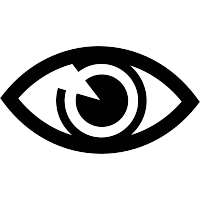High Resolution
(144 dpi)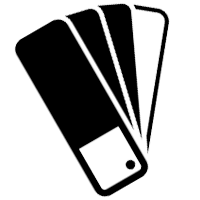Stunning Color & Clarity